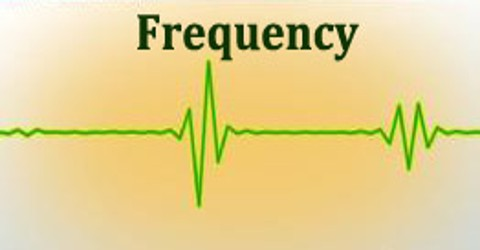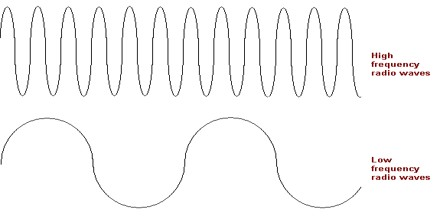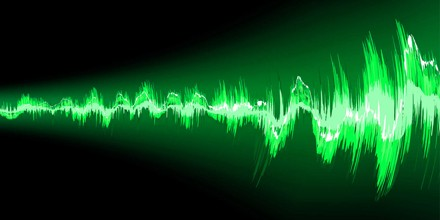Physics

# FrequencyFrequency

Definition

Frequency is the rate at which a repeating event occurs, such as the full cycle of a wave. Frequencies are usually measured in hertz. It is also referred to as temporal frequency, which emphasizes the contrast to spatial frequency and angular frequency. If a current completes one cycle per second, then the frequency is 1 Hz; 60 cycles per second equals 60 Hz, the standard alternating-current utility frequency in some countries.Frequency is a property of a wave and wave is a vibration that carries energy with it. It is an important parameter used in science and engineering to specify the rate of oscillatory and vibratory phenomena, such as mechanical vibrations, audio (sound) signals, radio waves, and light.

Frequency is also important in wireless communications, where the frequency of a signal is mathematically related to the wavelength. In physics and engineering disciplines, such as optics, acoustics, and radio, frequency is usually denoted by a Latin letter f or by the Greek letter ν or ν (nu). The frequency with which the Moon revolves around Earth is slightly more than 12 cycles per year. The frequency of the A string of a violin is 440 vibrations or cycles per second.Frequency Table

A frequency table is a chart that shows the popularity or mode of a certain type of data. When we look at a frequency, we are looking at the number of times an event occurs within a given scenario. A relative frequency table is a chart that shows the popularity or mode of a certain type of data based on the population sampled. When we look at relative frequency, we are looking at the number of times a specific event occurs compared to the total number of events.Symbols of Frequency

The symbols most often used for frequency are f and the Greek letters nu (ν) and omega (ω). Nu is used more often when specifying electromagnetic waves, such as light, X-rays, and gamma rays. Omega is usually used to describe the angular frequency that is, how much an object rotates or revolves in radians per unit time. Usually frequency is expressed in the hertz unit, named in honour of the 19th-century German physicist Heinrich Rudolf Hertz, one hertz being equal to one cycle per second, abbreviated Hz; one kilohertz (kHz) is 1,000 Hz, and one megahertz (MHz) is 1,000,000 Hz. In spectroscopy another unit of frequency, the wave number, is sometimes used.Frequency in Light and Sound

Light is an electromagnetic wave; it is a vibration in electric and magnetic fields. Because of this, it can travel through the vacuum of space, and thank goodness for that! If that wasn’t the case, we would get no heat from the sun, and life on Earth would be impossible. The frequency of the wave determines its color: 4×1014 Hz is red light, 8×1014 Hz is violet light, and between these (in the range 4-8×1014 Hz) are all the other colors of the visible spectrum. Our eyes detect the frequency of the light, and our brains interpret it as color.

Frequency is the property of sound that most determines pitch. The frequencies an ear can hear are limited to a specific range of frequencies. The audible frequency range for humans is typically given as being between about 20 Hz and 20,000 Hz (20 kHz), though the high frequency limit usually reduces with age. Other species have different hearing ranges. For example, some dog breeds can perceive vibrations up to 60,000 Hz.

Reference: study.com, britannica.com, wikipedia.